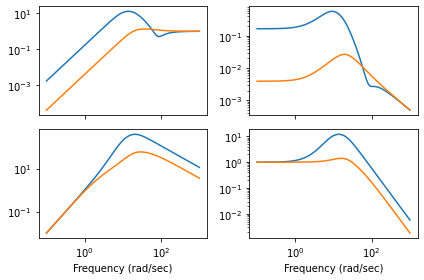# Vehicle steering¶

Karl J. Astrom and Richard M. Murray 23 Jul 2019

This notebook contains the computations for the vehicle steering running example in Feedback Systems.

:

import numpy as np
import matplotlib.pyplot as plt
import control as ct
import control.optimal as opt
ct.use_fbs_defaults()


## Vehicle steering dynamics (Example 3.11)¶

The vehicle dynamics are given by a simple bicycle model. We take the state of the system as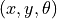where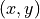is the position of the reference point of the vehicle in the plane and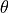is the angle of the vehicle with respect to horizontal. The vehicle input is given by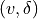where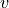is the forward velocity of the vehicle and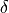is the angle of the steering wheel. We take as parameters the wheelbase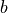and the offset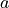between the rear wheels and the reference point. The model includes saturation of the vehicle steering angle (maxsteer).

• System state: x, y, theta

• System input: v, delta

• System output: x, y

• System parameters: wheelbase, refoffset, maxsteer

Assuming no slipping of the wheels, the motion of the vehicle is given by a rotation around a point O that depends on the steering angle. To compute the angle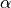of the velocity of the reference point with respect to the axis of the vehicle, we let the distance from the center of rotation O to the contact point of the rear wheel be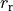and it the follows from Figure 3.17 in FBS that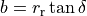and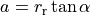, which implies that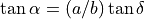.

Reasonable limits for the steering angle depend on the speed. The physical limit is given in our model as 0.5 radians (about 30 degrees). However, this limit is rarely possible when the car is driving since it would cause the tires to slide on the pavement. We us a limit of 0.1 radians (about 6 degrees) at 10 m/s (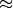35 kph) and 0.05 radians (about 3 degrees) at 30 m/s (110 kph). Note that a steering angle of 0.05 rad gives a cross acceleration of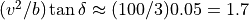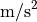at 10 m/s and 15at 30 m/s (1.5 times the force of gravity).

:

def vehicle_update(t, x, u, params):
# Get the parameters for the model
a = params.get('refoffset', 1.5)        # offset to vehicle reference point
b = params.get('wheelbase', 3.)         # vehicle wheelbase
maxsteer = params.get('maxsteer', 0.5)  # max steering angle (rad)

# Saturate the steering input
delta = np.clip(u, -maxsteer, maxsteer)
alpha = np.arctan2(a * np.tan(delta), b)

# Return the derivative of the state
return np.array([
u * np.cos(x + alpha),    # xdot = cos(theta + alpha) v
u * np.sin(x + alpha),    # ydot = sin(theta + alpha) v
(u / b) * np.tan(delta)      # thdot = v/l tan(phi)
])

def vehicle_output(t, x, u, params):
return x[0:2]

# Default vehicle parameters (including nominal velocity)
vehicle_params={'refoffset': 1.5, 'wheelbase': 3, 'velocity': 15,
'maxsteer': 0.5}

# Define the vehicle steering dynamics as an input/output system
vehicle = ct.NonlinearIOSystem(
vehicle_update, vehicle_output, states=3, name='vehicle',
inputs=('v', 'delta'), outputs=('x', 'y'), params=vehicle_params)


## Vehicle driving on a curvy road (Figure 8.6a)¶

To illustrate the dynamics of the system, we create an input that correspond to driving down a curvy road. This trajectory will be used in future simulations as a reference trajectory for estimation and control.

:

# System parameters
wheelbase = vehicle_params['wheelbase']
v0 = vehicle_params['velocity']

# Control inputs
T_curvy = np.linspace(0, 7, 500)
v_curvy = v0*np.ones(T_curvy.shape)
delta_curvy = 0.1*np.sin(T_curvy)*np.cos(4*T_curvy) + 0.0025*np.sin(T_curvy*np.pi/7)
u_curvy = [v_curvy, delta_curvy]
X0_curvy = [0, 0.8, 0]

# Simulate the system + estimator
t_curvy, y_curvy, x_curvy = ct.input_output_response(
vehicle, T_curvy, u_curvy, X0_curvy, params=vehicle_params, return_x=True)

# Configure matplotlib plots to be a bit bigger and optimize layout
plt.figure(figsize=[9, 4.5])

# Plot the resulting trajectory (and some road boundaries)
plt.subplot(1, 4, 2)
plt.plot(y_curvy, y_curvy)
plt.plot(y_curvy - 9/np.cos(x_curvy), y_curvy, 'k-', linewidth=1)
plt.plot(y_curvy - 3/np.cos(x_curvy), y_curvy, 'k--', linewidth=1)
plt.plot(y_curvy + 3/np.cos(x_curvy), y_curvy, 'k-', linewidth=1)

plt.xlabel('y [m]')
plt.ylabel('x [m]');
plt.axis('Equal')

# Plot the lateral position
plt.subplot(2, 2, 2)
plt.plot(t_curvy, y_curvy)
plt.ylabel('Lateral position $y$ [m]')

# Plot the steering angle
plt.subplot(2, 2, 4)
plt.plot(t_curvy, delta_curvy)
plt.ylabel('Steering angle $\\delta$ [rad]')
plt.xlabel('Time t [sec]')
plt.tight_layout()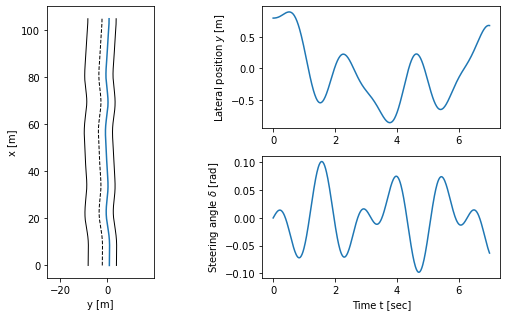## Linearization of lateral steering dynamics (Example 6.13)¶

We are interested in the motion of the vehicle about a straight-line path (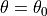) with constant velocity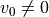. To find the relevant equilibrium point, we first set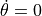and we see that we must have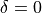, corresponding to the steering wheel being straight. The motion in the xy plane is by definition not at equilibrium and so we focus on lateral deviation of the vehicle from a straight line. For simplicity, we let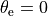, which corresponds to driving along the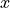axis. We can then focus on the equations of motion in the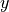anddirections with input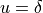.

:

# Define the lateral dynamics as a subset of the full vehicle steering dynamics
lateral = ct.NonlinearIOSystem(
lambda t, x, u, params: vehicle_update(
t, [0., x, x], [params.get('velocity', 1), u], params)[1:],
lambda t, x, u, params: vehicle_output(
t, [0., x, x], [params.get('velocity', 1), u], params)[1:],
states=2, name='lateral', inputs=('phi'), outputs=('y')
)

# Compute the linearization at velocity v0 = 15 m/sec
lateral_linearized = ct.linearize(lateral, [0, 0], , params=vehicle_params)

# Normalize dynamics using state [x1/b, x2] and timescale v0 t / b
b = vehicle_params['wheelbase']
v0 = vehicle_params['velocity']
lateral_transformed = ct.similarity_transform(
lateral_linearized, [[1/b, 0], [0, 1]], timescale=v0/b)

# Set the output to be the normalized state x1/b
lateral_normalized = lateral_transformed * (1/b)
print("Linearized system dynamics:\n")
print(lateral_normalized)

# Save the system matrices for later use
A = lateral_normalized.A
B = lateral_normalized.B
C = lateral_normalized.C

Linearized system dynamics:

A = [[0. 1.]
[0. 0.]]

B = [[0.5]
[1. ]]

C = [[1. 0.]]

D = [[0.]]



## Eigenvalue placement controller design (Example 7.4)¶

We want to design a controller that stabilizes the dynamics of the vehicle and tracks a given reference value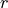of the lateral position of the vehicle. We use feedback to design the dynamics of the system to have the characteristic polynomial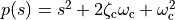.

To find reasonable values of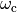we observe that the initial response of the steering angle to a unit step change in the steering command is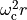, whereis the commanded lateral transition. Recall that the model is normalized so that the length unit is the wheelbaseand the time unit is the time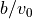to travel one wheelbase. A typical car has a wheelbase of about 3 m and, assuming a speed of 30 m/s, a normalized time unit corresponds to 0.1 s. To determine a reasonable steering angle when making a gentle lane change, we assume that the turning radius is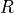= 600 m. For a wheelbase of 3 m this corresponds to a steering angle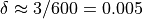rad and a lateral acceleration of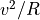= 302/600 = 1.5 m/s. Assuming that a lane change corresponds to a translation of one wheelbase we find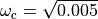= 0.07 rad/s.

The unit step responses for the closed loop system for different values of the design parameters are shown below. The effect ofis shown on the left, which shows that the response speed increases with increasing. All responses have overshoot less than 5% (15 cm), as indicated by the dashed lines. The settling times range from 30 to 60 normalized time units, which corresponds to about 3–6 s, and are limited by the acceptable lateral acceleration of the vehicle. The effect of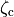is shown on the right. The response speed and the overshoot increase with decreasing damping. Using these plots, we conclude that a reasonable design choice is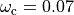and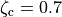.

:

# Utility function to place poles for the normalized vehicle steering system
def normalized_place(wc, zc):
# Get the dynamics and input matrices, for later use
A, B = lateral_normalized.A, lateral_normalized.B

# Compute the eigenvalues from the characteristic polynomial
eigs = np.roots([1, 2*zc*wc, wc**2])

# Compute the feedback gain using eigenvalue placement
K = ct.place_varga(A, B, eigs)

# Create a new system representing the closed loop response
clsys = ct.StateSpace(A - B @ K, B, lateral_normalized.C, 0)

# Compute the feedforward gain based on the zero frequency gain of the closed loop
kf = np.real(1/clsys(0))

# Scale the input by the feedforward gain
clsys *= kf

# Return gains and closed loop system dynamics
return K, kf, clsys

# Utility function to plot simulation results for normalized vehicle steering system
def normalized_plot(t, y, u, inpfig, outfig):
plt.sca(outfig)
plt.plot(t, y)
plt.sca(inpfig)
plt.plot(t, u)

# Utility function to label plots of normalized vehicle steering system
def normalized_label(inpfig, outfig):
plt.sca(inpfig)
plt.xlabel('Normalized time $v_0 t / b$')
plt.ylabel('Steering angle $\delta$ [rad]')

plt.sca(outfig)
plt.ylabel('Lateral position $y/b$')
plt.plot([0, 20], [0.95, 0.95], 'k--')
plt.plot([0, 20], [1.05, 1.05], 'k--')

# Configure matplotlib plots to be a bit bigger and optimize layout
plt.figure(figsize=[9, 4.5])

# Explore range of values for omega_c, with zeta_c = 0.7
outfig = plt.subplot(2, 2, 1)
inpfig = plt.subplot(2, 2, 3)
zc = 0.7
for wc in [0.5, 0.7, 1]:
# Place the poles of the system
K, kf, clsys = normalized_place(wc, zc)

# Compute the step response
t, y, x = ct.step_response(clsys, np.linspace(0, 20, 100), return_x=True)

# Compute the input used to generate the control response
u = -K @ x + kf * 1

# Plot the results
normalized_plot(t, y, u, inpfig, outfig)

# Add labels to the figure
normalized_label(inpfig, outfig)
plt.legend(('$\omega_c = 0.5$', '$\omega_c = 0.7$', '$\omega_c = 0.1$'))

# Explore range of values for zeta_c, with omega_c = 0.07
outfig = plt.subplot(2, 2, 2)
inpfig = plt.subplot(2, 2, 4)
wc = 0.7
for zc in [0.5, 0.7, 1]:
# Place the poles of the system
K, kf, clsys = normalized_place(wc, zc)

# Compute the step response
t, y, x = ct.step_response(clsys, np.linspace(0, 20, 100), return_x=True)

# Compute the input used to generate the control response
u = -K @ x + kf * 1

# Plot the results
normalized_plot(t, y, u, inpfig, outfig)

# Add labels to the figure
normalized_label(inpfig, outfig)
plt.legend(('$\zeta_c = 0.5$', '$\zeta_c = 0.7$', '$\zeta_c = 1$'))
plt.tight_layout()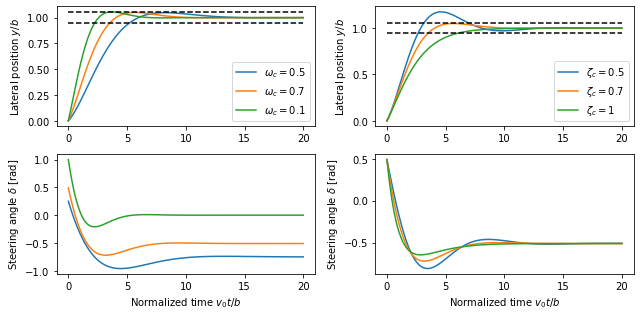## Eigenvalue placement observer design (Example 8.3)¶

We construct an estimator for the (normalized) lateral dynamics by assigning the eigenvalues of the estimator dynamics to desired value, specifified in terms of the second order characteristic equation for the estimator dynamics.

:

# Find the eigenvalue from the characteristic polynomial
wo = 1          # bandwidth for the observer
zo = 0.7        # damping ratio for the observer
eigs = np.roots([1, 2*zo*wo, wo**2])

# Compute the estimator gain using eigenvalue placement
L = np.transpose(
ct.place(np.transpose(A), np.transpose(C), eigs))
print("L = ", L)

# Create a linear model of the lateral dynamics driving the estimator
est = ct.StateSpace(A - L @ C, np.block([[B, L]]), np.eye(2), np.zeros((2,2)))

L =  [[1.4]
[1. ]]


### Linear observer applied to nonlinear system output¶

A simulation of the observer for a vehicle driving on a curvy road is shown below. The first figure shows the trajectory of the vehicle on the road, as viewed from above. The response of the observer is shown on the right, where time is normalized to the vehicle length. We see that the observer error settles in about 4 vehicle lengths.

:

# Convert the curvy trajectory into normalized coordinates
x_ref = x_curvy / wheelbase
y_ref = x_curvy / wheelbase
theta_ref = x_curvy
tau = v0 * T_curvy / b

# Simulate the estimator, with a small initial error in y position
t, y_est, x_est = ct.forced_response(est, tau, [delta_curvy, y_ref], [0.5, 0], return_x=True)

# Configure matplotlib plots to be a bit bigger and optimize layout
plt.figure(figsize=[9, 4.5])

# Plot the actual and estimated states
ax = plt.subplot(2, 2, 1)
plt.plot(t, y_ref)
plt.plot(t, x_est)
ax.set(xlim=[0, 10])
plt.legend(['actual', 'estimated'])
plt.ylabel('Lateral position $y/b$')

ax = plt.subplot(2, 2, 2)
plt.plot(t, x_est - y_ref)
ax.set(xlim=[0, 10])
plt.ylabel('Lateral error')

ax = plt.subplot(2, 2, 3)
plt.plot(t, theta_ref)
plt.plot(t, x_est)
ax.set(xlim=[0, 10])
plt.xlabel('Normalized time $v_0 t / b$')
plt.ylabel('Vehicle angle $\\theta$')

ax = plt.subplot(2, 2, 4)
plt.plot(t, x_est - theta_ref)
ax.set(xlim=[0, 10])
plt.xlabel('Normalized time $v_0 t / b$')
plt.ylabel('Angle error')
plt.tight_layout()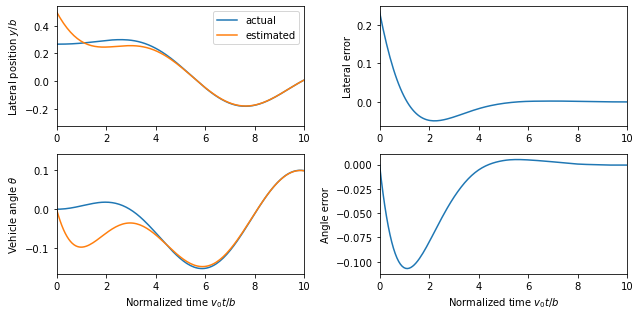## Output Feedback Controller (Example 8.4)¶

:

# Compute the feedback gains
# K, kf, clsys = normalized_place(1, 0.707)     # Gains from MATLAB
# K, kf, clsys = normalized_place(0.07, 0.707)  # Original gains
K, kf, clsys = normalized_place(0.7, 0.707)       # Final gains

# Print out the gains
print("K = ", K)
print("kf = ", kf)

# Construct an output-based controller for the system
clsys = ct.StateSpace(
np.block([[A, -B@K], [L@C, A - B@K - L@C]]),
np.block([[B], [B]]) * kf,
np.block([[C, np.zeros(C.shape)], [np.zeros(C.shape), C]]),
np.zeros((2,1)))

# Simulate the system
t, y, x = ct.forced_response(clsys, tau, y_ref, [0.4, 0, 0.0, 0], return_x=True)

# Calcaluate the input used to generate the control response
u_sfb = kf * y_ref - K @ x[0:2]
u_ofb = kf * y_ref - K @ x[2:4]

# Configure matplotlib plots to be a bit bigger and optimize layout
plt.figure(figsize=[9, 4.5])

# Plot the actual and estimated states
ax = plt.subplot(1, 2, 1)
plt.plot(t, x)
plt.plot(t, x)
plt.plot(t, y_ref, 'k-.')
ax.set(xlim=[0, 30])
plt.legend(['state feedback', 'output feedback', 'reference'])
plt.xlabel('Normalized time $v_0 t / b$')
plt.ylabel('Lateral position $y/b$')

ax = plt.subplot(2, 2, 2)
plt.plot(t, x)
plt.plot(t, x)
plt.plot(t, theta_ref, 'k-.')
ax.set(xlim=[0, 15])
plt.ylabel('Vehicle angle $\\theta$')

ax = plt.subplot(2, 2, 4)
plt.plot(t, u_sfb)
plt.plot(t, u_ofb)
plt.plot(t, delta_curvy, 'k-.')
ax.set(xlim=[0, 15])
plt.xlabel('Normalized time $v_0 t / b$')
plt.ylabel('Steering angle $\\delta$')
plt.tight_layout()

K =  [[0.49   0.7448]]
kf =  0.4899999999999182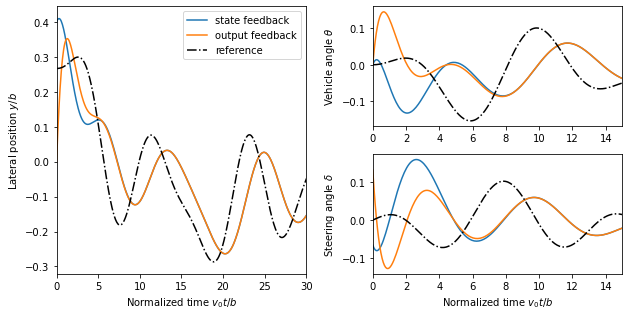## Trajectory Generation (Example 8.8)¶

To illustrate how we can use a two degree-of-freedom design to improve the performance of the system, consider the problem of steering a car to change lanes on a road. We use the non-normalized form of the dynamics, which were derived in Example 3.11.

:

import control.flatsys as fs

# Function to take states, inputs and return the flat flag
def vehicle_flat_forward(x, u, params={}):
# Get the parameter values
b = params.get('wheelbase', 3.)

# Create a list of arrays to store the flat output and its derivatives
zflag = [np.zeros(3), np.zeros(3)]

# Flat output is the x, y position of the rear wheels
zflag = x
zflag = x

# First derivatives of the flat output
zflag = u * np.cos(x)  # dx/dt
zflag = u * np.sin(x)  # dy/dt

# First derivative of the angle
thdot = (u/b) * np.tan(u)

# Second derivatives of the flat output (setting vdot = 0)
zflag = -u * thdot * np.sin(x)
zflag =  u * thdot * np.cos(x)

return zflag

# Function to take the flat flag and return states, inputs
def vehicle_flat_reverse(zflag, params={}):
# Get the parameter values
b = params.get('wheelbase', 3.)

# Create a vector to store the state and inputs
x = np.zeros(3)
u = np.zeros(2)

# Given the flat variables, solve for the state
x = zflag  # x position
x = zflag  # y position
x = np.arctan2(zflag, zflag)  # tan(theta) = ydot/xdot

# And next solve for the inputs
u = zflag * np.cos(x) + zflag * np.sin(x)
thdot_v = zflag * np.cos(x) - zflag * np.sin(x)
u = np.arctan2(thdot_v, u**2 / b)

return x, u

vehicle_flat = fs.FlatSystem(vehicle_flat_forward, vehicle_flat_reverse, inputs=2, states=3)

:

# Utility function to plot lane change trajectory
def plot_vehicle_lanechange(traj):
# Create the trajectory
t = np.linspace(0, Tf, 100)
x, u = traj.eval(t)

# Configure matplotlib plots to be a bit bigger and optimize layout
plt.figure(figsize=[9, 4.5])

# Plot the trajectory in xy coordinate
plt.subplot(1, 4, 2)
plt.plot(x, x)
plt.xlabel('y [m]')
plt.ylabel('x [m]')

# Add lane lines and scale the axis
plt.plot([-4, -4], [0, x[0, -1]], 'k-', linewidth=1)
plt.plot([0, 0], [0, x[0, -1]], 'k--', linewidth=1)
plt.plot([4, 4], [0, x[0, -1]], 'k-', linewidth=1)
plt.axis([-10, 10, -5, x[0, -1] + 5])

# Time traces of the state and input
plt.subplot(2, 4, 3)
plt.plot(t, x)
plt.ylabel('y [m]')

plt.subplot(2, 4, 4)
plt.plot(t, x)

plt.subplot(2, 4, 7)
plt.plot(t, u)
plt.xlabel('Time t [sec]')
plt.ylabel('v [m/s]')
# plt.axis([0, t[-1], u0 - 1, uf + 1])

plt.subplot(2, 4, 8)
plt.plot(t, u);
plt.xlabel('Time t [sec]')
plt.ylabel('$\delta$ [rad]')
plt.tight_layout()


To find a trajectory from an initial state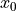to a final state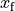in time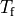we solve a point-to-point trajectory generation problem. We also set the initial and final inputs, which sets the vehicle velocityand steering wheel angleat the endpoints.

:

# Define the endpoints of the trajectory
x0 = [0., 2., 0.]; u0 = [15, 0.]
xf = [75, -2., 0.]; uf = [15, 0.]
Tf = xf / uf

# Define a set of basis functions to use for the trajectories
poly = fs.PolyFamily(8)

# Find a trajectory between the initial condition and the final condition
traj1 = fs.point_to_point(vehicle_flat, Tf, x0, u0, xf, uf, basis=poly)
plot_vehicle_lanechange(traj1)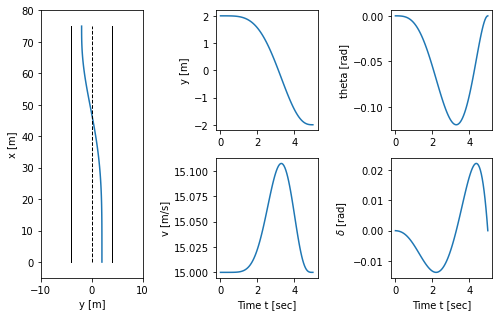### Change of basis function¶

:

bezier = fs.BezierFamily(8)
traj2 = fs.point_to_point(vehicle_flat, Tf, x0, u0, xf, uf, basis=bezier)
plot_vehicle_lanechange(traj2)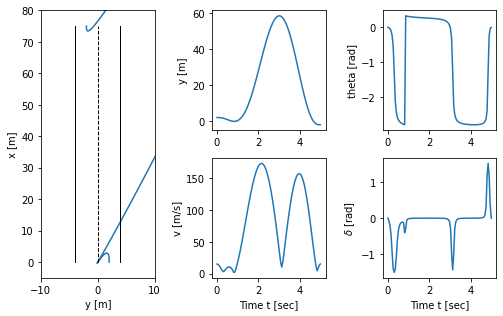:

timepts = np.linspace(0, Tf, 12)
poly = fs.PolyFamily(8)
vehicle_flat, np.diag([0, 0.1, 0]), np.diag([0.1, 10]), x0=xf, u0=uf)
constraints = [
opt.input_range_constraint(vehicle_flat, [8, -0.1], [12, 0.1]) ]

traj3 = fs.point_to_point(
vehicle_flat, timepts, x0, u0, xf, uf, cost=traj_cost, basis=poly
)
plot_vehicle_lanechange(traj3)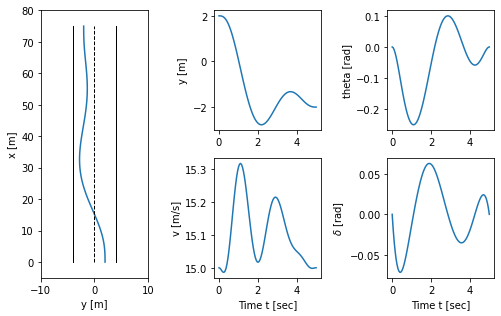## Vehicle transfer functions for forward and reverse driving (Example 10.11)¶

The vehicle steering model has different properties depending on whether we are driving forward or in reverse. The figures below show step responses from steering angle to lateral translation for a the linearized model when driving forward (dashed) and reverse (solid). In this simulation we have added an extra pole with the time constant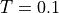to approximately account for the dynamics in the steering system.

With rear-wheel steering the center of mass first moves in the wrong direction and the overall response with rear-wheel steering is significantly delayed compared with that for front-wheel steering. (b) Frequency response for driving forward (dashed) and reverse (solid). Notice that the gain curves are identical, but the phase curve for driving in reverse has non-minimum phase.

:

# Magnitude of the steering input (half maximum)
Msteer = vehicle_params['maxsteer'] / 2

# Create a linearized model of the system going forward at 2 m/s
forward_lateral = ct.linearize(lateral, [0, 0], , params={'velocity': 2})
forward_tf = ct.ss2tf(forward_lateral)[0, 0]
print("Forward TF = ", forward_tf)

# Create a linearized model of the system going in reverise at 1 m/s
reverse_lateral = ct.linearize(lateral, [0, 0], , params={'velocity': -2})
reverse_tf = ct.ss2tf(reverse_lateral)[0, 0]
print("Reverse TF = ", reverse_tf)

Forward TF =
s + 1.333
-----------------------------
s^2 + 7.828e-16 s - 1.848e-16

Reverse TF =
-s + 1.333
-----------------------------
s^2 - 7.828e-16 s - 1.848e-16


:

# Configure matplotlib plots to be a bit bigger and optimize layout
plt.figure()

# Forward motion
t, y = ct.step_response(forward_tf * Msteer, np.linspace(0, 4, 500))
plt.plot(t, y, 'b--')

# Reverse motion
t, y = ct.step_response(reverse_tf * Msteer, np.linspace(0, 4, 500))
plt.plot(t, y, 'b-')

# Add labels and reference lines
plt.axis([0, 4, -0.5, 2.5])
plt.legend(['forward', 'reverse'], loc='upper left')
plt.xlabel('Time $t$ [s]')
plt.ylabel('Lateral position [m]')
plt.plot([0, 4], [0, 0], 'k-', linewidth=1)

# Plot the Bode plots
plt.figure()
plt.subplot(1, 2, 2)
ct.bode_plot(forward_tf[0, 0], np.logspace(-1, 1, 100), color='b', linestyle='--')
ct.bode_plot(reverse_tf[0, 0], np.logspace(-1, 1, 100), color='b', linestyle='-')
plt.legend(('forward', 'reverse'));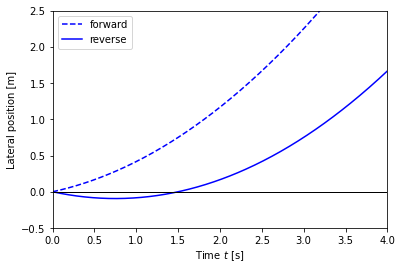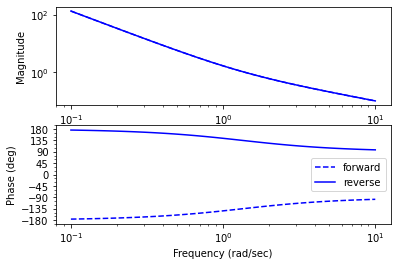## Feedforward Compensation (Example 12.6)¶

For a lane transfer system we would like to have a nice response without overshoot, and we therefore consider the use of feedforward compensation to provide a reference trajectory for the closed loop system. We choose the desired response as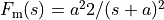, where the response speed or aggressiveness of the steering is governed by the parameter.

:

# Define the desired response of the system
a = 0.2
P = ct.ss2tf(lateral_normalized)
Fm = ct.TransferFunction([a**2], [1, 2*a, a**2])
Fr = Fm / P

# Compute the step response of the feedforward components
t, y_ffwd = ct.step_response(Fm, np.linspace(0, 25, 100))
t, delta_ffwd = ct.step_response(Fr, np.linspace(0, 25, 100))

# Scale and shift to correspond to lane change (-2 to +2)
y_ffwd = 0.5 - 1 * y_ffwd
delta_ffwd *= 1

plt.subplot(1, 2, 1)
plt.plot(y_ffwd, t)
plt.plot(-1*np.ones(t.shape), t, 'k-', linewidth=1)
plt.plot(0*np.ones(t.shape), t, 'k--', linewidth=1)
plt.plot(1*np.ones(t.shape), t, 'k-', linewidth=1)
plt.axis([-5, 5, -2, 27])

# Plot the response
plt.subplot(2, 2, 2)
plt.plot(t, y_ffwd)
# plt.axis([0, 10, -5, 5])
plt.ylabel('Normalized position y/b')

plt.subplot(2, 2, 4)
plt.plot(t, delta_ffwd)
# plt.axis([0, 10, -1, 1])
plt.ylabel('$\\delta$ [rad]')
plt.xlabel('Normalized time $v_0 t / b$');

plt.tight_layout()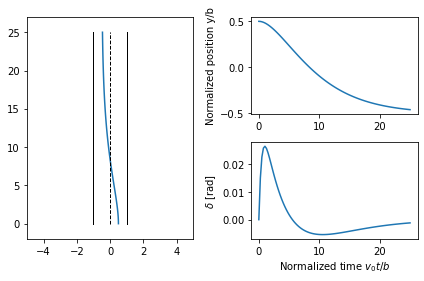## Fundamental Limits (Example 14.13)¶

Consider a controller based on state feedback combined with an observer where we want a faster closed loop system and choose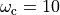,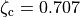,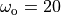, and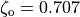.

:

# Compute the feedback gain using eigenvalue placement
wc = 10
zc = 0.707
eigs = np.roots([1, 2*zc*wc, wc**2])
K = ct.place(A, B, eigs)
kr = np.real(1/clsys(0))
print("K = ", np.squeeze(K))

# Compute the estimator gain using eigenvalue placement
wo = 20
zo = 0.707
eigs = np.roots([1, 2*zo*wo, wo**2])
L = np.transpose(
ct.place(np.transpose(A), np.transpose(C), eigs))
print("L = ", np.squeeze(L))

# Construct an output-based controller for the system
C1 = ct.ss2tf(ct.StateSpace(A - B@K - L@C, L, K, 0))
print("C(s) = ", C1)

# Compute the loop transfer function and plot Nyquist, Bode
L1 = P * C1
plt.figure(); ct.nyquist_plot(L1, np.logspace(0.5, 3, 500))
plt.figure(); ct.bode_plot(L1, np.logspace(-1, 3, 500));

K =  [100.   -35.86]
L =  [ 28.28 400.  ]
C(s) =
-1.152e+04 s + 4e+04
--------------------
s^2 + 42.42 s + 6658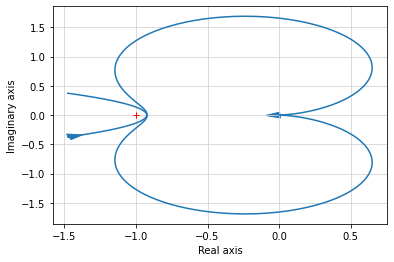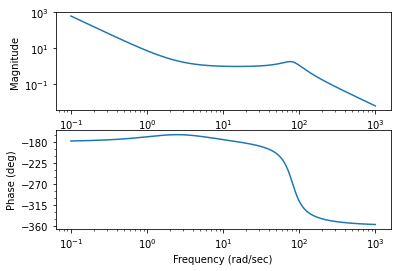:

# Modified control law
wc = 10
zc = 2.6
eigs = np.roots([1, 2*zc*wc, wc**2])
K = ct.place(A, B, eigs)
kr = np.real(1/clsys(0))
print("K = ", np.squeeze(K))

# Construct an output-based controller for the system
C2 = ct.ss2tf(ct.StateSpace(A - B@K - L@C, L, K, 0))
print("C(s) = ", C2)

K =  [100.   2.]
C(s) =
3628 s + 4e+04
---------------------
s^2 + 80.28 s + 156.6


:

# Plot the gang of four for the two designs
ct.gangof4(P, C1, np.logspace(-1, 3, 100))
ct.gangof4(P, C2, np.logspace(-1, 3, 100))